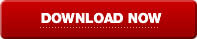# Fractional Odds Explained

Fractional odds are a method of demonstrating odds which are inclusive of your profit and exclusive of your stake - to get your total return you need to add your stake back in. This system is used more frequently in the UK; in the US this is typically used when reporting bets at future events.

An example of Fractional Odds:

A £5 stake at 6/4 = £7.50 (Profit) plus your stake = £12.50 total return.

Converting fractional odds to decimal places is as simple as dividing the left hand figure by the right hand figure and adding 1.

An example of converting fractional odds into decimals:

6/4 + 1 =1.5 +1= 2.5 (therefore £10 x 2.5 = £25.00 = your Total Return).